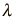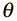Bragg's LawWhen x-rays are scattered from a crystal lattice, peaks of scattered intensity are observed which correspond to the following conditions:

1. The angle of incidence = angle of scattering.
2. The pathlength difference is equal to an integer number of wavelengths.

The condition for maximum intensity contained in Bragg's law above allow us to calculate details about the crystal structure, or if the crystal structure is known, to determine the wavelength of the x-rays incident upon the crystal.Click on active symbol above to perform calculation

 For a wavelength= nm and order n =,

 a lattice spacing of d = nm

 would give a diffraction maximum at= degrees.

This calculation is designed to calculate wavelength, crystal plane separation or diffraction angle. After entering data, click on the symbol of the quantity you wish to calculate in the active graphic above. Default data will be entered for any unspecified quantity, but all values can be changed.

 Bragg spectrometer Characteristic x-rays
 Brillouin scattering: application to light scattering
Index

Reference
Thornton & Rex
Sec. 6.1

 HyperPhysics***** Quantum Physics R Nave
Go Back

Bragg Spectrometer

Much of our knowledge about crystal structure and the structure of molecules as complex as DNA in crystalline form comes from the use of x-rays in x-ray diffraction studies. A basic instrument for such study is the Bragg spectrometer.To obtain nearly monochromatic x-rays, an x-ray tube is used to produce characteristic x-rays. In order to eliminate as much of the brehmsstrahlung continuum radiation as possible, matched filters are used in the x-ray beam to optimize the fraction of the energy which is in the K-alpha line. Such filters use elements just above and just below the metal in the x-ray target, making use of the strong "absorption edges" just above and below the K-alpha energy of the target metal.

The x-rays are collimated with apertures in a strong x-ray absorber (usually lead) and the narrow resulting x-ray beam is allowed to strike the crystal to be studied. The spectrometer arrangement couples the rotation of the crystal with the rotation of the detector so that the angle of rotation of the detector is twice that of the crystal. This satisfies the conditions of Bragg's law for diffraction of the x-rays from the crystal lattice planes.

 Application in Compton scattering
Index

 HyperPhysics***** Quantum Physics R Nave
Go Back